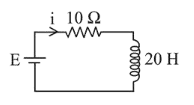# A 20 Henry inductor coil is connected to a 10 ohm resistance in series as shown in figure.Question:

A 20 Henry inductor coil is connected to a 10 ohm resistance in series as shown in figure. The time at which rate of dissipation of energy (Joule's heat) across resistance is equal to the rate at which magnetic energy is stored in the inductor, is :1. (1) $\frac{2}{\ln 2}$

2. (2) $\frac{1}{2} \ln 2$

3. (3) $2 \ln 2$

4. (4) $\ln 2$

Correct Option: 3,

Solution:

(3) $i^{2} R=\left(\tau \frac{d i}{d t}\right) i$

$\Rightarrow \frac{d i}{d t}=\frac{i}{\tau}$

$\Rightarrow \mathrm{t}=\tau \ln 2=2 \ln 2\left[\right.$ as $\left.\tau=\frac{L}{R}=\frac{20}{10}=2\right]$